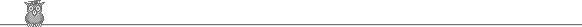### Putnam 1992Problem A2

Let the coefficient of x1992 in the power series (1 + x)α = 1 + αx + ... be C(α). Find ∫01 C(-y-1) ∑k=119921/(y+k) dy.

Solution

Trivial.

The usual Taylor series gives C(-y-1) = (y+1)(y+2) ... (y+1992)/1992! . Hence the integrand is simply the derivative of (y+1)(y+2) ... (y+1992)/1992! , so the integral evaluates to 1993 - 1 = 1992.